# 2.8 – Expected Value, Variance, Standard Deviation

Previous: 2.7 – A Geometric Problem

Next: 2.9 – Example

Analogous to the discrete case, we can define the expected value, variance, and standard deviation of a continuous random variable. These quantities have the same interpretation as in the discrete setting. The expectation of a random variable is a measure of the centre of the distribution, its mean value. The variance and standard deviation are measures of the horizontal spread or dispersion of the random variable.

Definition: Expected Value, Variance, and Standard Deviation of a Continuous Random Variable
The expected value of a continuous random variable X, with probability density function f(x), is the number given byThe variance of X is: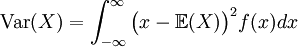As in the discrete case, the standard deviation, σ, is the positive square root of the variance: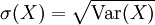## Animation

The following animation encapsulates the concepts of the CDF, PDF, expected value, and standard deviation of a normal random variable. When viewing the animation, it may help to remember that

• the "mean" is another term for expected value
• the standard deviation is equal to the positive square root of the variance
• the CDF (lower plot) is an antiderivative of the PDF (upper plot)

Connecting the CDF and the PDF (requires the Wolfram "CDF Player")

## Simple Example

The random variable X is given by the following PDF. Check that this is a valid PDF and calculate the standard deviation of X.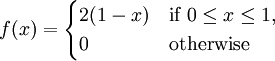### Solution

#### Part 1

To verify that f(x) is a valid PDF, we must check that it is everywhere nonnegative and that it integrates to 1.

We see that 2(1-x) = 2 - 2x ≥ 0 precisely when x ≤ 1; thus f(x) is everywhere nonnegative.

To check that f(x) has unit area under its graph, we calculateSo f(x) is indeed a valid PDF.

#### Part 2

To calculate the standard deviation of X, we must first find its variance. Calculating the variance of X requires its expected value: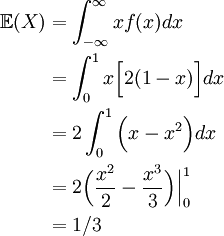Using this value, we compute the variance of X as follows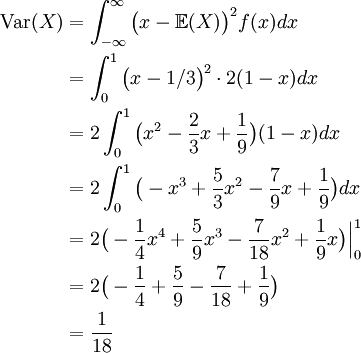Therefore, the standard deviation of X is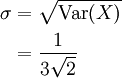### An Alternative Formula for Variance

There is an alternative formula for the variance of a random variable that is less tedious than the above definition.

Alternate Formula for the Variance of a Continuous Random Variable
The variance of a continuous random variable X with PDF f(x) is the number given by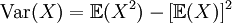The derivation of this formula is a simple exercise and has been relegated to the exercises. We should note that a completely analogous formula holds for the variance of a discrete random variable, with the integral signs replaced by sums.

## Simple Example Revisited

We can use this alternate formula for variance to find the standard deviation of the random variable X defined above.

Remembering that E(X) was found to be 1/3, we compute the variance of X as follows: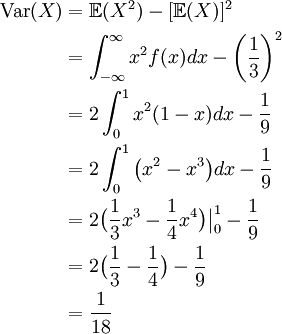In the exercises, you will compute the expectations, variances and standard deviations of many of the random variables we have introduced in this chapter, as well as those of many new ones.

Previous: 2.7 – A Geometric Problem

Next: 2.9 – Example